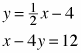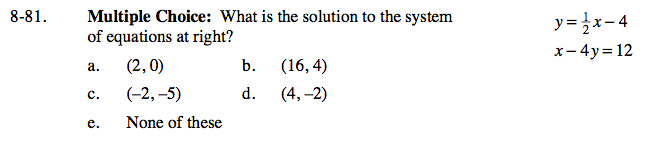Home > GC > Chapter 8 > Lesson 8.2.2 > Problem8-81

8-81.

Multiple Choice: What is the solution to the system of equations below? Homework Help ✎a. (2, 0) b. (16, 4) c. (−2, −5) d. (4, −2) None of theseUse substitution to solve for x and y.

(d)